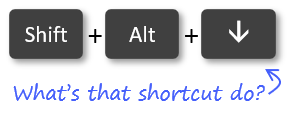# Excel VBA Code to Reference Column Numbers Instead of Letters

Often times we want to reference a column with numbers instead of letters in VBA.  The following macro contains various examples of how to reference one or more columns by the column number in Excel.

This is great if you are basing the column reference on a formula result, variable, or cell's value.

```Sub Column_Number_References()
'This macro contains VBA examples of how to
'reference or select a column by numbers instead of letters

Dim lCol As Long

'The standard way to reference columns by letters
Range("A:B").Select
Range("A:A").Select
Columns("A").Select
Columns("A:F").Select

'Select a single column with Columns property
'The following line selects column B (column 2)
Columns(2).Select

'Select columns based on a variable
lCol = 2
Columns(lCol).Select

'Select columns based on variable of a cell's value
'Cell A1 must contain a valid column number.
lCol = Range("A1").Value
Columns(lCol).Select

'Select multiple columns with the Range and Columns properties
'The following line selects columns B:E
Range(Columns(2), Columns(5)).Select

'Select multiple columns with the Columns and Resize properties
'The following line selects columns B:D.
'It starts a column 2 and resizes the range by 3 columns.
'The row parameter of the resize property is left blank.
'This is an optional parameter (argument) and is
'not required for the column resize.
Columns(2).Resize(, 3).Select

End Sub
```

•Victor says:

Hi Jon, this info has help me a lot.
Thanks
Victor

•Jon Acampora says:

Awesome! Thanks so much for letting me know Victor. 🙂

•John-Paul says:

I visited ten websites before finding this – this was exactly what I was looking for. Super helpful

•Jon Acampora says:

Thank you John-Paul. I really appreciate your support.

•Bobby says:

Super helpful, so many sites weren’t.

•HAFIZ ARMAN says:

HELLO
I LIKED THIS AND WAS VERY HELPFUL FOR ME
THANKS A LOT OF

•Øyvind Hansen says:

Thanks a lot mate.

•apsis says:

Little gem… “Columns(2).Resize(, 3).Select” Many thanks!

•Isaac says:

Very quick & informative solution! Thank you!!

•Junko says:

Thank you for the comments on range cells.
I’m trying to delete multiple columns at once time but it comes with error : compile error (sub and function not defined)

n = “5_8_2019_AUS”
With Worksheet(n)
.Range(.Columns(4), .Columns(6)).Delete
End With

•Abhijat says:

Many thanks for this. It is indeed very helpful. I was wondering if there is a similar code to select non-continuous columns .. for example how will one select column 1, 3 and 5 at the same time using a vba code.

•Abhijat says:

To add to my comment, I found a solution. Here it is for your review
The question was : How to select multiple non-continuous columns.

Union(Range(Columns(1), Columns(1)), Range(Columns(3), Columns(3)), Range(Columns(pucFirstColumnQtr – 1), Columns(pucFirstColumnQtr – 1)), Range(Columns(pucLastColumnQtr + 1), Columns(pucLastColumnQtr + 1))).Select

•gvg says:

Range(Columns(2), Columns(3)).Select – this doesn’t select strictly columns B:C if you have a merged cell between B:D somewhere .

•js says:

I want to use VBA to set a Conditional Formatting rule. I know the start column of the Rule (Column 2) but the end column is dynamic. I use a code line to find the column number where I need to end:
Stopp = Range(“A4:CM4″).Find(what:=”stop”, LookAt:=xlWhole).Column

I am then trying the following to inject the Rule:

With Worksheets(“GGG”).Range(Columns(2) & “:” & Columns(stopp)).FormatConditions.Add(Type:=xlExpression, Formula1:=””)

…but it is not populating.

Can you help?

Generic filters
Exact matches only

#### Excel Shortcuts ListLearn over 270 Excel keyboard & mouse shortcuts for Windows & Mac.

Excel Shortcuts List